Share filterDeveloped by in Tools category

4/5.0 Ratings

5,000 - 10,000 Installs

81 Reviews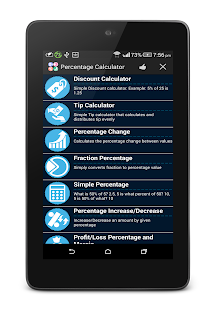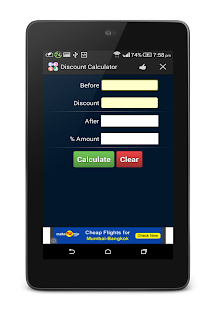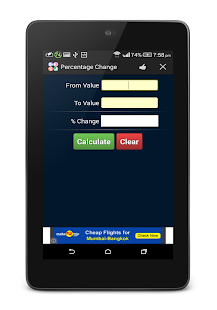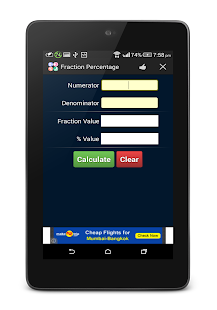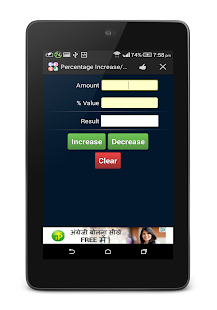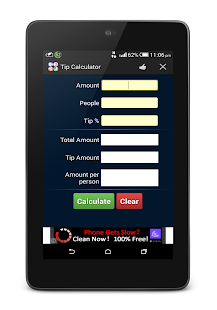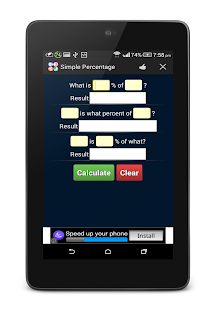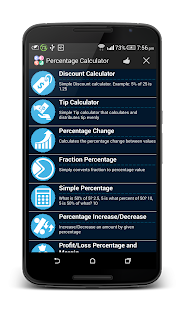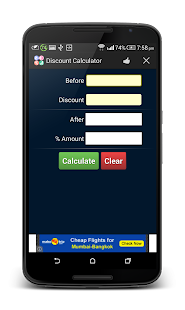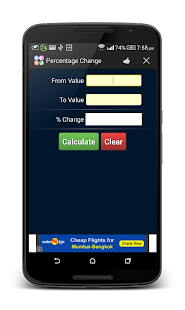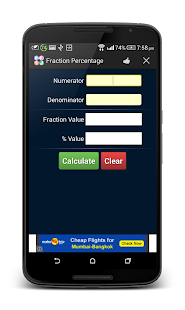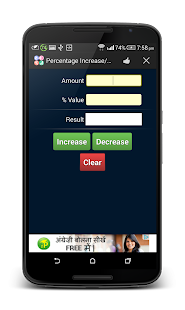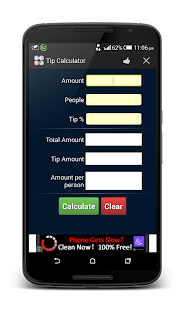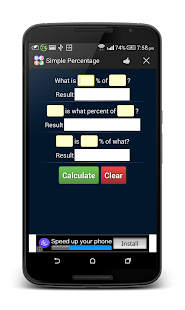### Description

Percentage Calculator is a quick and simple app which calculates:

* Discounts
* Restaurant tip - calculated and distributes tip evenly
* Percentage change - calculates the percentage change between two values
* Fraction percentage - simply converts fraction to percentage
* Simple percentage - performs simple percentage calculation like 5 is what percent of 50? 10
* Percentage increase/decrease - Increase/decrease an amount by given percentage
* Profit/loss percentage and margin - calculates profit/loss percentage as a percentage of cost and profit/loss margin as a percentage of revenue.

Features:
* Simple, quick and responsive UI
* Tablet support
* Available in multiple languages